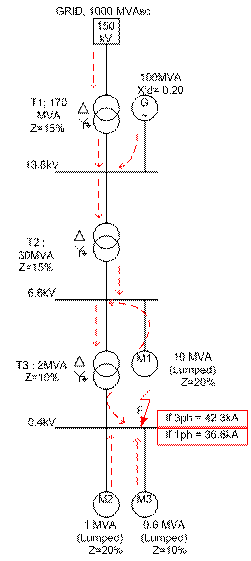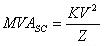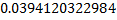Short circuit calculation using MVA method (manual)

By : Gusti  Anggara,
Maaf saya  tulis dalam bahasa inggris, karena ini saya belajar dari literatur berbahasa inggris.Utility: 150KV, 1000 MVAsc
Transformer 1: 170 MVA, 150/13.8KV, 15% Z

13.8KV Bus
Generator: 100MVA, X"d = 0.2
Transformer 2: 30 MVA, 13.8/6.6KV, 15% Z

6.6KV Bus
Transformer 3: 2MVA, 6.6KV/400V, 10% Z
Motor 1: 10 MVA (Lumped), 20% Z

400V Bus
Motor2: 1000 KVA (Lumped), 20% Z
Motor 3: 600 KVA (Lumped), 10% Z

In the event of ashort circuit, thesources of short circuit current are
1. Utility
2. Generators
3. Motors

Static loads such as heaters and lighting do not contributeto short circuit.
"EquivalentMVA" are:
Transformers and MotorsGeneratorsCables and ReactorsSo, here are the results of MVAsc:
Utility: MVAsc = 1000MVA
Transformer 1: MVAsc = 170 / 0.15 = 1133.33 MVA

13.8KV Bus
Generator: MVAsc = 100 / 0.2 = 500 MVA
Transnformer 2: MVAsc = 30 / 0.15 = 200 MVA

6.6KV Bus
Transformer 3: MVAsc = 2 / 0.1 = 20 MVA
Motor 1: MVAsc = 10 / 0.2 = 50 MVA

400V Bus
Motor 2: MVAsc = 1 / 0.2 = 5 MVA
Motor 3: MVAsc = 0.6 / 0.1 = 6 MVA

Now we calculate the upstream contribution :
At Transformer 1:
MVAsc @ 150KV = 1000 MVA
MVAsc @ 13.8KV = 1/ (1 / 1000 + 1 /1133.33) = 531.25 MVA

At Transformer 2:
MVAsc @ 13.8KV = 531.25 + 500 = 1031.25 MVA
MVAsc @ 6.6KV = 1/ (1 / 1031.25 + 1 / 200) = 167.51 MVA

At Transformer 3:
MVAsc @ 6.6KV = 167.51 + 50 = 217.51 MVA
MVAsc @ 400V = 1/ (1 / 217.51 + 1 / 20) = 18.31 MVA

At 400V Motors
Motor 3: MVAsc = 18.31 x 5 / ( 5 + 6 ) = 8.3 MVA
Motor 4: MVAsc = 18.31 x 6 / ( 5 + 6 ) = 9.98 MVA
The fault MVAsc @bus 400V = 18.31 + 5 + 6 = 29.31MVAsc
The three phase  If = 29.31/(1.732*(0.4)) = 42.3 kA.

Now we come to fault single phase to ground :
For single phase faults, positive sequence, negative sequence and zero sequence
impedances need to be calculated.
If = 3 (I1 + I2 + I0)

Examining the circuit in above, at the 400V Bus, on Transformer 3 contributes to the
zero sequence current.

For transformers, the negative sequence and zero sequence impedance are equal to the positive sequence impedance.

Z1 = Z2 = Z0 or
MVA1 = MVA2 = MVA0
@bus 400V;
1 / MVAsc =1/3 (1 / MVAsc1 + 1 / MVAsc2 + 1 / MVAsc0)
1 / MVAsc = 1/3 (1 / 29.31 + 1 / 29.31 + 1 / 20 )
1/ MVAsc =MVAsc = 1/= 25.4 MVAsc

If= 25.4 / (1.732 x 0.4) = 36.6 kA

This post first appeared on Dunia Listrik, please read the originial post: here

Share the post

Short circuit calculation using MVA method (manual)

×